﻿ Math Expert

Back to start page

Math Expert

1. Introduction

The application "math expert" is a collection of formulas from mathematics and physics. The special feature is that the application can calculate the formulas. The calculation is based on the motto "Tell me what you know, I am looking which calculations are possible."

The formulas are divided into categories and subcategories. The following list is intended to illustrate this:

• Math
• Basic arithmetic
• Area calculation
• Circle
• Rectangle
• etc.
• etc.
• Physics
• Others
• Favorites

In the example would be "math," the category, "Area Calculation" the subcategory and "circle" or "rectangle" are the corresponding formulas.

The category of "favorites" has a special meaning. The user ia able to add or remove existing formulas to this category.

With the menu "Search" you can find a formula.

For certain formulas you can visualize the function graph.

2. Calculate formulas

In the introduction we already mentioned that the formulas are assigned to categories and subcategories. Categories and subcategories are presented in lists. By selecting a list entry, you go into the next screen. With the "Back" button you can switch to the previous screen.

The form for entering a formula consists of at least one input field and one output field.

A "dot" is always the decimal separator.

The formula is calculated by the button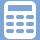in the navigation bar. It is now attempting, with the input variables to calculate the remaining variables.

If the entered values are not plausible, the application display an error message ("... The existing value and calculated value doesn't match ...").

2.1 General

In the toolbar, the following buttons are displayed depending on availability:

Button DescriptionThe calculation will be performed. This button is in the navigation bar.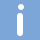The button "Details" describes the individual parameters. Sometimes even more detailed information on the formula and to the computation path are available.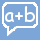You can see how the calculation was made. It should be noted that the numerical values are displayed with the settings of display precision.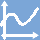This button is visible, if a function graph for the formula is available.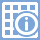If additional information are available, for example a list of specific resistances, they can use this button to view.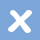With this button you can delete the values of the form.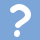Shows this app documentation.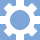You can change system settings.

2.2 Computation path

The menu item/toolbar button "Computation path" you can see how the calculation was made. It should be noted that the numerical values are displayed with the settings of display precision.

A formula can have auxiliary variables. These variables are visible in the computation path, i.e. they do not appear as an input field and are not described in the help page.

2.3 Settings

2.3.1 Calculation precision

All calculations are done with the precision of decimal digits given here.

If the numerical values are very small, then the precision can be adjusted accordingly. The error message "... The existing value and calculated value ... do not match", eg indicate an insufficient precision of the calculation.

2.3.2 Display precision

The results are shown with the display precision.

If a number is rounded, it is shown in italics. The calculation is done with the unrounded numbers.

2.3.3 Angular dimension

The following angular dimension are supported:

• DEG (Grad): 0..360°
• RAD (Radiant): 0..2 · π
• GRAD (Neugrad): 0..400gon

2.3.4 Scientific view

To be able to read large or small numbers easier, there is the view of numbers in the "Scientific Presentation". The numbers can be visualized as a power with multiples of three. Corresponds for example 100000 = 100.0E3 = 100k.

2.3.5 Number of input fields

The application should run on as many different platforms. For this reason, the user can specify the number of input fields of a line. For very narrow or wide screen and/or in very large numbers, you can adjust this setting.

3. Functions graph

The function graph of a formula starts with the button "function graph". The application attempts the x-and y-coordinates to be adjusted so that the most interesting area is shown. About the settings, the coordinates are adapted, too.

4. Contact/Support

The formulary is not yet complete and will be expanded in the coming weeks.

Errors and problems can be sent to the following e-mail: math-expert@ejbguru.de.

We are at the above address also always grateful for constructive criticism and suggestions.

The Math Expert Team wishes you much fun with the application.

Back to start page